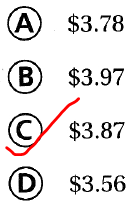# Texas Go Math Grade 5 Lesson 1.8 Answer Key Add and Subtract Money

Refer to our Texas Go Math Grade 5 Answer Key Pdf to score good marks in the exams. Test yourself by practicing the problems from Texas Go Math Grade 5 Lesson 1.8 Answer Key Add and Subtract Money.

## Texas Go Math Grade 5 Lesson 1.8 Answer Key Add and Subtract Money

Unlock the Problem

At the end of May, Mrs. Freeman had an account balance of $442.37. Since then, she has written a check for$63.92 and made a deposit of $350.00. Mrs. Freeman says she has$729.45 in her account. Make a table to determine if Mrs. Freeman is correct.

What do I need to find?
I need to find the amount she had in each month

What information am I given?
I am given the initial amount of money in May’s account and the final amount of money in May’s account

Plan

What is my plan or strategy?
I need to make a table and use the information to find the amount of money May has in each month

Solve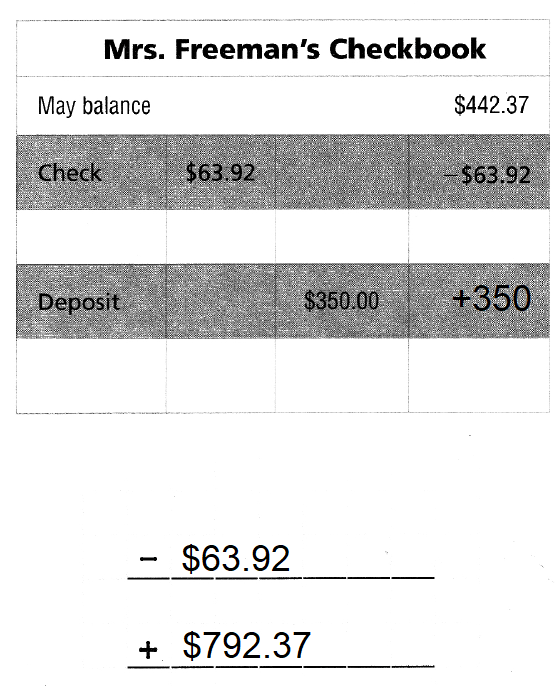So,
Mrs. Freeman’s correct balance is $728.45 Question 1. How can you tell if your answer is reasonable? Answer: Check the answer by subtracting the amount May has in each month from$728.45.
If we get $442.37, then your answer is reasonable Try Another Problem Nick is buying juice for himself and 5 friends. Each bottle of juice costs$1.25. How much do 6 bottles of juice cost? Make a table to find the cost of 6 bottles of juice.

What do I need to find?
I need to find the total cost of 6 bottles of juice

What information am I given?
I am given the cost of each bottle of juice and the number of persons

Plan

What is my plan or strategy?
Multiply the number of persons with the cost of each bottle of juice

Solve

So, the total cost of 6 bottles of juice is $7.5 Question 2. What if Ginny says that 12 bottles of juice cost$25.00? Is Ginny’s statement reasonable? Explain.
It is given that
Ginny says that 12 bottles of juice cost $25.00 Now, From the above problem, We can observe that The cost of 6 bottles of juice is:$7.5
The cost of each bottle of juice is: $1.25 Now, The cost of 12 bottles of juice = 12 ×$1.25
= 6 × 2 × $1.25 =$7.5 × 2
= $15 Hence, from the above, We can conclude that Ginny’s statement is not reasonable Question 3. If Nick had$10, how many bottles of juice could he buy?
It is given that
Nick had $10 Now, From the above problem, We can observe that Each bottle of juice costs$1.25
Now,
The number of bottles of juice Nick can buy = (The amount of money Nick had) ÷ (The cost of each bottle of juice)
= $$\frac{10}{1.25}$$
= 8 bottles
Hence, from the above,
We can conclude that
Nick can buy 8 bottles of juice with $10 Math Talk Mathematical Processes Explain how you could use another strategy to solve the problem. Answer: We can solve a problem either by a multiplication or division strategy In multiplication strategy, We will consider the unknown as x and find the result In division strategy, We will consider the unknown and solve it by using the multiplication strategy Share and Show Question 1. Sara wants to buy a bottle of apple juice from a vending machine. She needs exactly$2.30. She has the following bills and coins: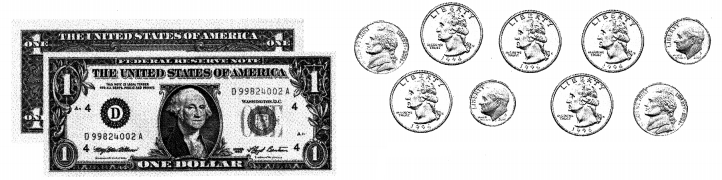Make and complete a table to find all the ways Sara could pay for the juice.
First, draw a table with a column for each type of bill or coin.
Next, fill in your table with each row showing a different way Sara can make exactly $2.30. Answer: It is given that Sara wants to buy a bottle of apple juice from a vending machine. She needs exactly$2.30.
So,
The complete table that shows the arrangement of different ways that Sara can make $2.30 is: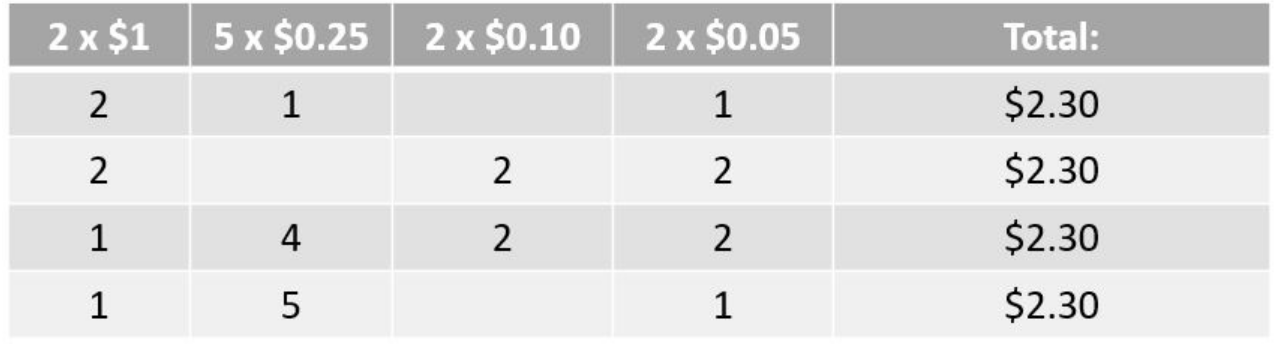Hence, from the above, We can conclude that The completed table shows the different ways Sara can make exactly$2.30 is:Question 2.
What if Sara decides to buy a bottle of water that costs $1.85? What are all the different ways she can make exactly$1.85 with the bills and coins she has? Which coin must Sara use?
It is given that
Sara decides to buy a bottle of water that costs $1.85 Now, The different ways that Sara can make exactly$1.85 is:
a.
1 bill, 3 quarters, 1 dime
So,
$1 + 3 x$0.25 + $0.10 =$1.85
b.
1 bill, 3 quarters, 2 nickels;
So,
$1 + 3 x$0.25 + 2 x $0.05 =$1.85
So,
Sara must use “Quarter”
Hence, from the above,
We can conclude that
Sara must use “Quarter”

Problem Solving

Use the following Information to solve 3-4.

At Open Skate Night, admission is $3.75 with a membership card and$5.00 without a membership card. Skate rentals are $3.00. Question 3. H.O.T. Multi-Step The Moores paid$6 more for skate rentals than the Cotters did. Together, the two families paid $30 for skate rentals. How many pairs of skates did the Moores rent? Answer: It is given that At Open Skate Night, admission is$3.75 with a membership card and $5.00 without a membership card. Skate rentals are$3.00.
The Moores paid $6 more for skate rentals than the Cotters did. Together, the two families paid$30 for skate rentals
Now,
Let the amount paid by Cotters be: $x So, The amount paid by Moores for Skate rentals is:$(x + 6)
So,
According to the given information,
x + x + 6 = $30 2x +$6 = $30 2x =$24
x = $$\frac{24}{2}$$
x = $12 So, The amount paid by Cotters for skate rentals is:$12
The amount paid by Moores for skate rentals is: $18 Now, Let the pairs of skates Moores own be: x So, The number of pairs of skates Moores rent = $$\frac{18}{3}$$ = 6 pairs Hence, from the above, We can conclude that The number of pairs of skates Moores rents is: 6 pairs Question 4. H.O.T. Multi-Step Jennie and 5 of her friends are going to Open Skate Night. Jennie does not have a membership card. Only some of her friends have membership cards. What is the total amount that Jennie and her friends might pay for admission? Answer: It is given that Jennie and 5 of her friends are going to Open Skate Night. Jennie does not have a membership card. Only some of her friends have membership cards At Open Skate Night, admission is$3.75 with a membership card and $5.00 without a membership card. Skate rentals are$3.00.
So,
According to the given information,
The total amount that Jennie and her friends might pay for admission = $5 + 5 ($3.75)
= $5 +$18.75
= $23.75 Hence, from the above, We can conclude that The total amount that Jennie and her friends might pay for admission is:$23.75

Fill in the bubble completely to show your answer.

Question 5.
Apply Sherry and Mario want to buy a pack of pencils to split for the new school year. The pack of pencils costs $3.68. They each have$1.25 to spend. How much more money do they need to buy the pencils?
A. $1.18 B.$2.50
C. $2.43 D.$4.93
It is given that
Sherry and Mario want to buy a pack of pencils to split for the new school year. The pack of pencils costs $3.68. They each have$1.25 to spend.
So,
The total amount of money they have to spend = 2 × $1.25 =$2.5
So,
The amount of more money do they need to buy the pencils = $3.68 –$2.5
= $1.18 Hence, from the above, We can conclude that The amount of more money do they need to buy the pencils is: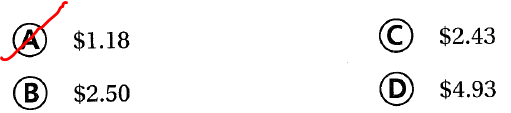Question 6. Analyze Marc has one dollar, one quarter, one dime, one nickel, and one penny. He spends 35 cents. How much money does he have left? A.$0.76
B. $0.96 C.$1.06
D. $1.16 Answer: It is given that Marc has one dollar, one quarter, one dime, one nickel, and one penny. He spends 35 cents. So, The amount of money Marc has left = ($1 + $0.25 + 0.10 +$0.05 + $0.01) –$0.35
= $1.41 –$0.35
= $1.06 Hence, from the above, We can conclude that The amount of money that Marc has left is:Question 7. Multi-Step Maria bought two cups of milk for$1.09 each, and a salad for $7.75. If she pays with a ten-dollar bill, how much change does she receive? A.$0.07
B. $1.16 C.$2.25
D. $7.82 Answer: It is given that Maria bought two cups of milk for$1.09 each, and a salad for $7.75 and she pays with a ten-dollar bill So, The change does Mara will receive =$10 – (2 ($1.09) +$7.75)
= $10 –$9.93
= $0.07 Hence, from the above, We can conclude that The change that Mara will receive is:Texas Test Prep Question 8. Sean and Hope are going bowling. For each person, a game costs$4.25. Shoe rentals are $3.00. Sean gives the clerk$20 for their admission and shoe rental. How much change should he receive?
A. $12.75 B.$5.50
C. $9.75 D.$14.50
It is given that
Sean and Hope are going bowling. For each person, a game costs $4.25. Shoe rentals are$3.00. Sean gives the clerk $20 for their admission and shoe rental. So, The total cost of a game =$4.25 + $3.00 =$7.25
So,
The total cost of a game for 2 persons = 2 ($7.25) =$14.5
So,
The change should Sean receive = $20 –$14.50
= $5.5 Hence, from the above, We can conclude that The change should Sean receive is: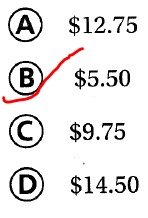### Texas Go Math Grade 5 Lesson 1.8 Homework and Practice Answer Key Question 1. Sam wants to buy a snack from a vending machine. He needs exactly$3.20. Complete the table to find the different ways Sam could pay for the snack.It is given that
Sam wants to buy a snack from a vending machine. He needs exactly $3.20. So, The complete table that shows the different ways Sam could pay for the snack is: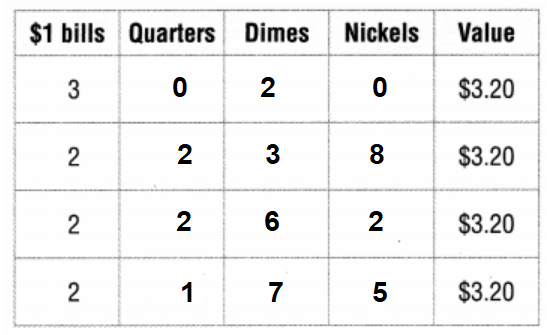Hence, from the above, We can conclude that The completed table shows the different ways Sam could pay for the snack. is:Question 2. Mandy is buying popcorn for herself and 5 friends. Each bag of popcorn costs$0.95. How much do 6 bags of popcorn cost? Complete the table to find the total cost.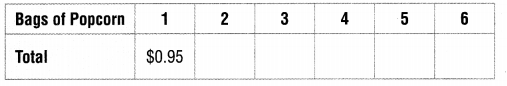It is given that
Mandy is buying popcorn for herself and 5 friends. Each bag of popcorn costs $0.95 Now, The given table is:So, According to the given information, The completed table is: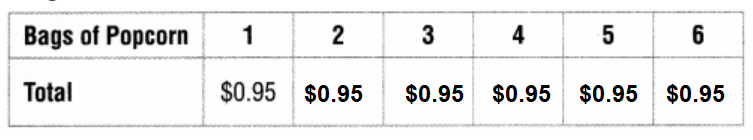So, The cost of 6 bags of popcorn = 6 ×$0.95
= $5.7 Hence, from the above, We can conclude that The cost of 6 bags of popcorn is:$5.70

Question 3.
Orlando is buying 6 bottles of water. Each bottle of water costs $1.19. How much do 6 bottles of water cost? Complete the table to find the total cost.Answer: It is given that Orlando is buying 6 bottles of water. Each bottle of water costs$1.19
Now,
The given table is:So,
According to the given information,
The completed table is: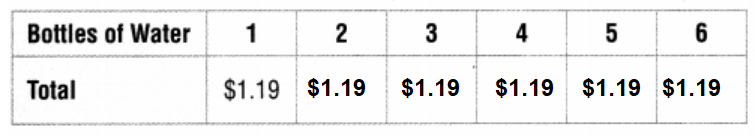So,
The total cost of 6 bottles of water = 6 × $1.19 =$7.14
Hence, from the above,
We can conclude that
The total cost of 6 bottles of water is: $7.14 Problem Solving Question 4. At the end of September, Ms. Diaz had an account balance of$623.41. Since then, she has written checks for $149.00 and$27.50 and made a deposit of $299.00. Ms. Diaz says her balance is$845.91. Find Ms. Diaz’s correct balance.It is given that
At the end of September, Ms. Diaz had an account balance of $623.41. Since then, she has written checks for$149.00 and $27.50 and made a deposit of$299.00. Ms. Diaz says her balance is $845.91. So, The amount of money she has at present after deposits and written checks =$299 – ($149 +$27.50)
= $122.50 So, The total amount of money i.e, her balance money in the account =$623.41 + $122.50 =$745.91
Hence, from the above,
We can conclude that
Ms. Diaz’s correct balance is: $745.91 Lesson Check Fill in the bubble completely to show your answer. Question 5. One shirt costs$7.90. What is the price of 4 shirts?
A. $31.60 B.$28.36
C. $32.10 D.$31.42
It is given that
One shirt costs $7.90 So, The cost of 4 shirts = 4 ×$7.90
= $31.60 Hence, from the above, We can conclude that The cost of 4 shirts is: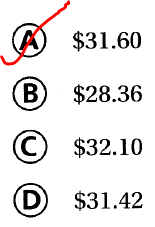Question 6. Multi-Step Jorge’s checking account balance is$458.00 He writes a check for $89.20 and donates$20.00 to a local charity. Then he makes a deposit of $92.19. What is his new checking account total? A.$440.99
B. $398.80 C.$480.99
D. $256.61 Answer: It is given that Jorge’s checking account balance is$458.00 He writes a check for $89.20 and donates$20.00 to a local charity. Then he makes a deposit of $92.19 So, Jorge’s new checking account total =$458 + ($92.19 – ($89.20 + $20.00)) =$458 – $17.01 =$440.99
Hence, from the above,
We can conclude that
Jorge’s new checking account total is:Question 7.
Multi-Step Theodore buys a sandwich for $4.47 and two salads for$5.10 each. If he pays with a $20 bill, how much change does he receive? A.$5.24
B. $5.21 C.$5.19
D. $5.33 Answer: It is given that Theodore buys a sandwich for$4.47 and two salads for $5.10 each and he pays with a$20 bill
So,
The change does Theodore received = $20 – ($4.47 + 2 ($5.10)) =$5.33
Hence, from the above,
We can conclude that
The change does Theodore received is: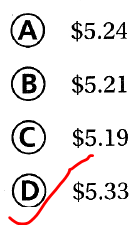Question 8.
Multi-Step Carleen has three quarters, four dimes, and three nickels. She spends 55 cents. How much money does she have left?
A. $0.80 B.$0.75
C. $0.60 D.$0.85
It is given that
Carleen has three quarters, four dimes, and three nickels. She spends 55 cents
So,
The money does Carleen has left = (3 × 0.25 + 4 × 0.10 + 3 × 0.05) – $0.55 = (0.75 + 0.40 + 0.15) –$0.55
= $0.75 Hence, from the above, We can conclude that The money does Carleen has left is: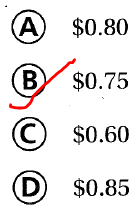Question 9. Multi-Step Mr. Lee has a balance of$237.35 after he wrote two checks. One check was for $350.40 and the other was for$211.25. What was his balance before he wrote the checks?
A. $597 B.$799
C. $561 D.$780
It is given that
Mr. Lee has a balance of $237.35 after he wrote two checks. One check was for$350.40 and the other was for $211.25 So, The balance of Mr. Lee before he wrote the checks =$237.35 + ($350.40 +$211.25)
= $799 Hence, from the above, We can conclude that The balance of Mr. Lee before he wrote the checks is: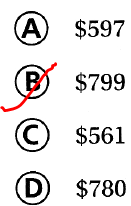Question 10. Multi-Step Andrew bought balloons for his dad’s birthday for$5.30. The tax on the balloons is $0.83. If he pays with a$10 bill, how much change does he receive?
A. $3.78 B.$3.97
C. $3.87 D.$3.56
Andrew bought balloons for his dad’s birthday for $5.30. The tax on the balloons is$0.83. If he pays with a $10 bill So, The change does Andrew received =$10 – ($5.30 +$0.83)